# 1 Getting started

The sechm package is a wrapper around the ComplexHeatmap package to facilitate the creation of annotated heatmaps from objects of the Bioconductor class SummarizedExperiment (and extensions thereof).

## 1.1 Package installation

``````if (!requireNamespace("BiocManager", quietly = TRUE))
install.packages("BiocManager")
BiocManager::install("sechm")``````

## 1.2 Example data

To showcase the main functions, we will use an example object which contains (a subset of) RNAseq of mouse hippocampi after Forskolin-induced long-term potentiation:

``````suppressPackageStartupMessages({
library(SummarizedExperiment)
library(sechm)
})
data("Chen2017", package="sechm")
SE <- Chen2017``````

This is taken from Chen et al., 2017.

# 2 Example usage

## 2.1 Basic functionalities

The `sechm` function simplifies the generation of heatmaps from `SummarizedExperiment`. It minimally requires, as input, a `SummarizedExperiment` object and a set of genes (or features, i.e. rows of `sechm`) to plot:

``````g <- c("Egr1", "Nr4a1", "Fos", "Egr2", "Sgk1", "Arc", "Dusp1", "Fosb", "Sik1")
sechm(SE, features=g)``````
``## Using assay logFC``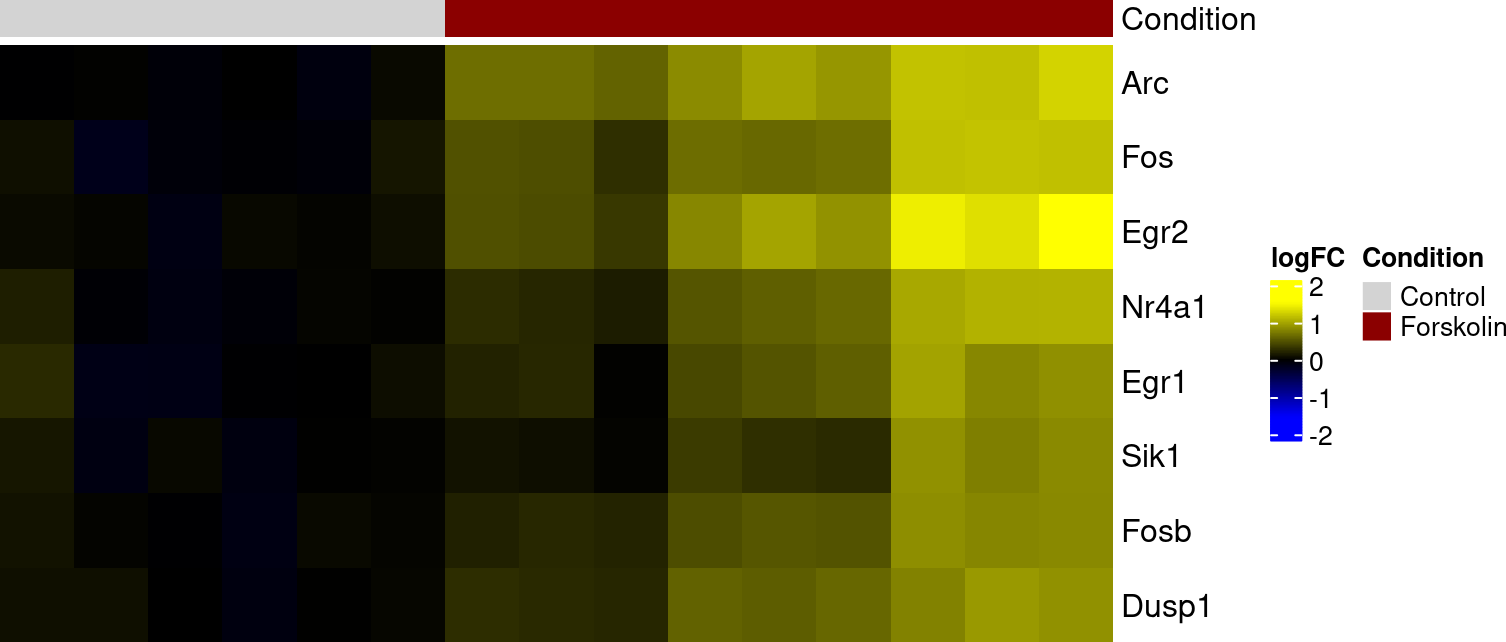``````# with row scaling:
sechm(SE, features=g, do.scale=TRUE)``````
``## Using assay logFC``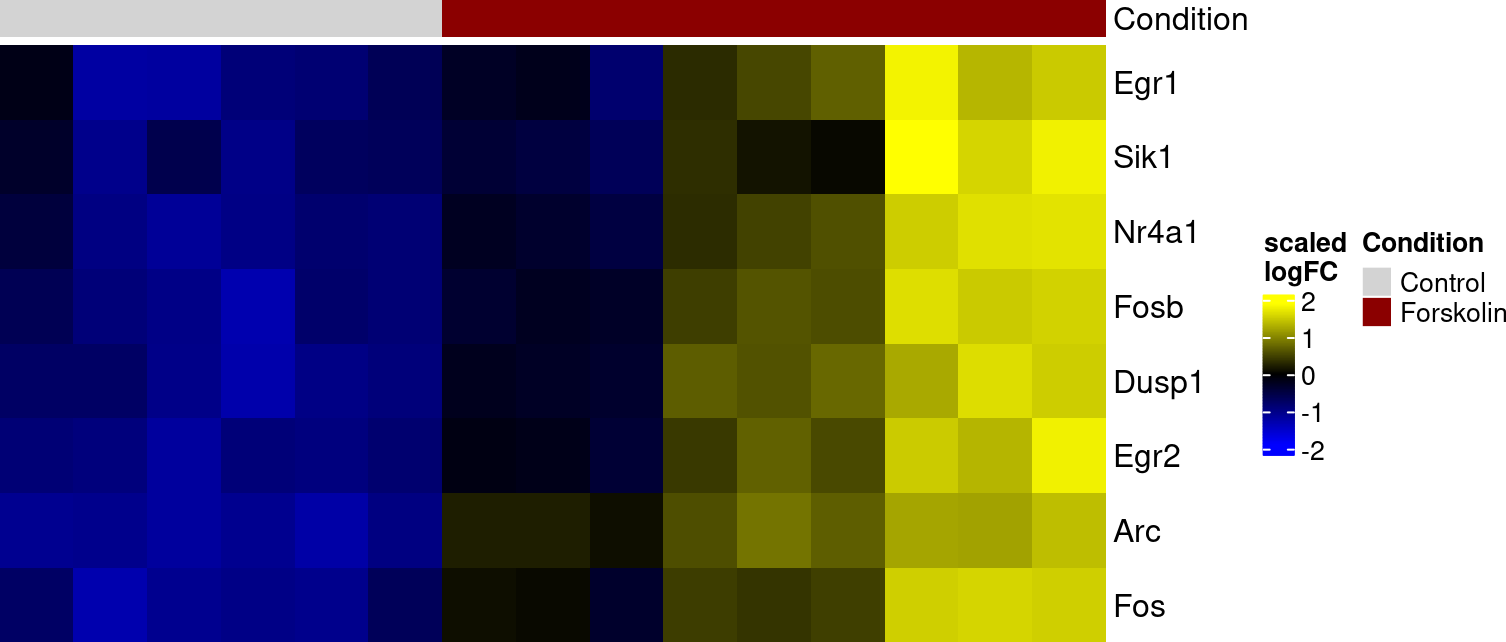The assay can be selected, and any `rowData` or `colData` columns can be specified as annotation:

``````rowData(SE)\$meanLogCPM <- rowMeans(assays(SE)\$logcpm)
sechm(SE, features=g, assayName="logFC", top_annotation=c("Condition","Time"), left_annotation=c("meanLogCPM"))``````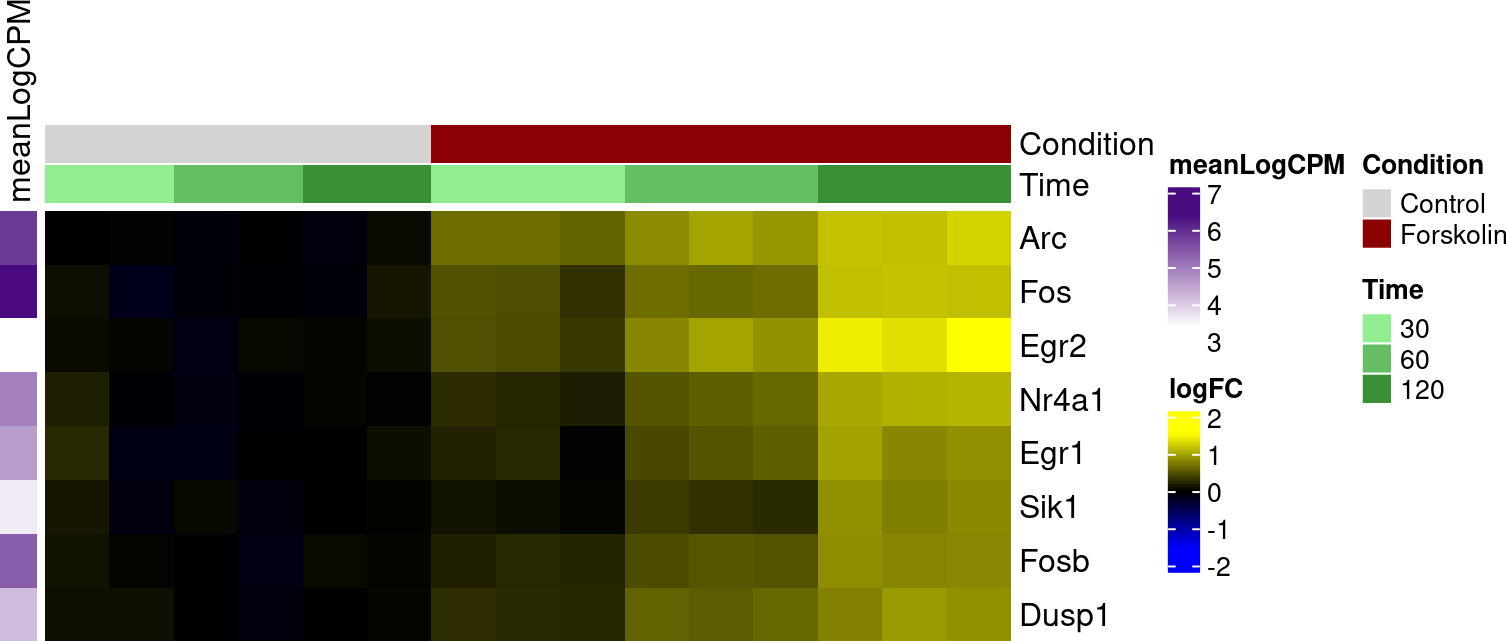Column names are ommitted by default, but can be displayed:

``sechm(SE, features=g, do.scale=TRUE, show_colnames=TRUE)``
``## Using assay logFC``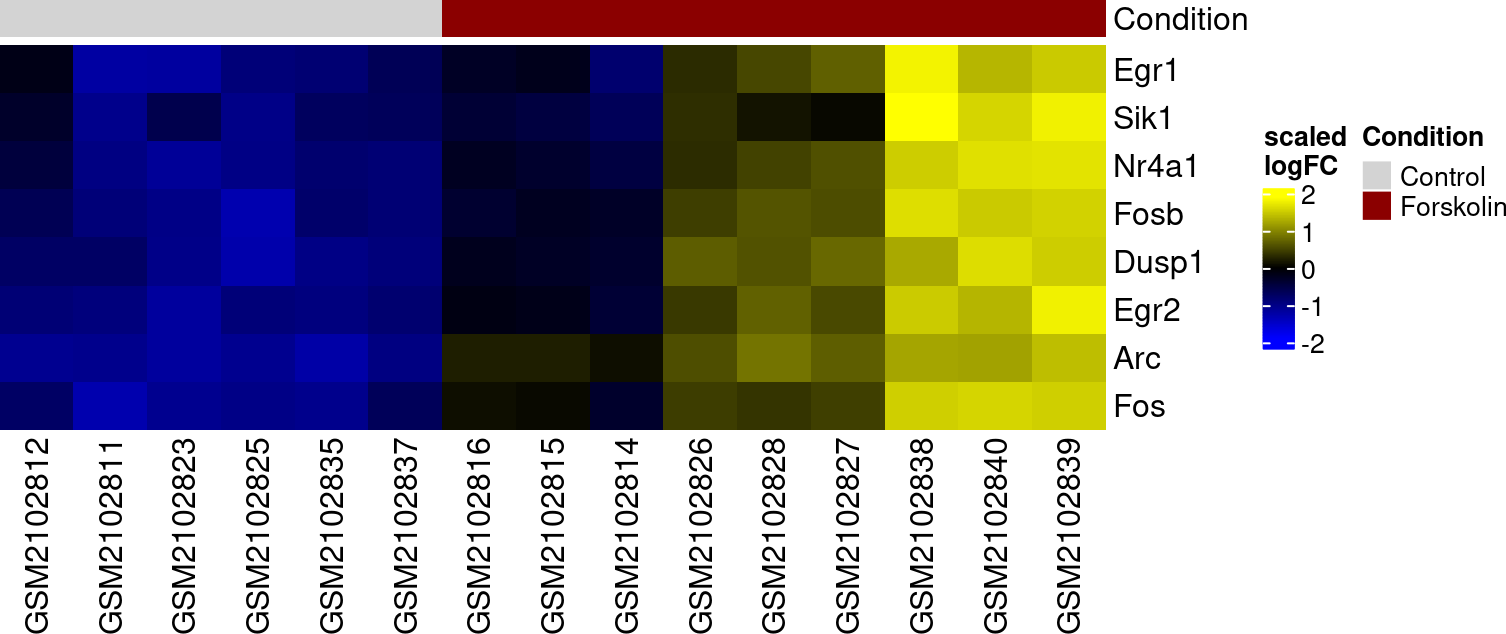Since `sechm` uses the ComplexHeatmap engine for plotting, any argument of `ComplexHeatmap::Heatmap` can be passed:

``sechm(SE, features=g, do.scale=TRUE, row_title="My genes")``
``## Using assay logFC``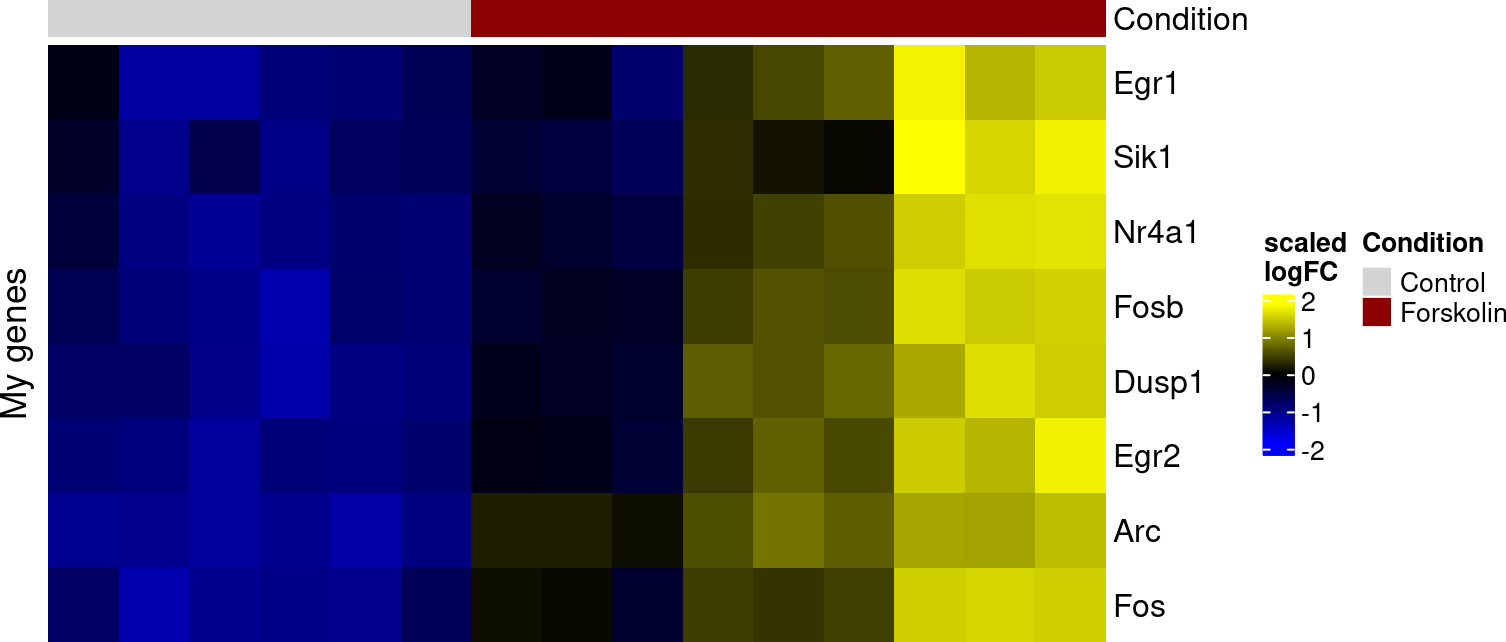When plotting a lot of rows, by default row names are not shown (can be overriden), but specific genes can be highlighted with the `mark` argument:

``sechm(SE, features=row.names(SE), mark=g, do.scale=TRUE, top_annotation=c("Condition","Time"))``
``## Using assay logFC``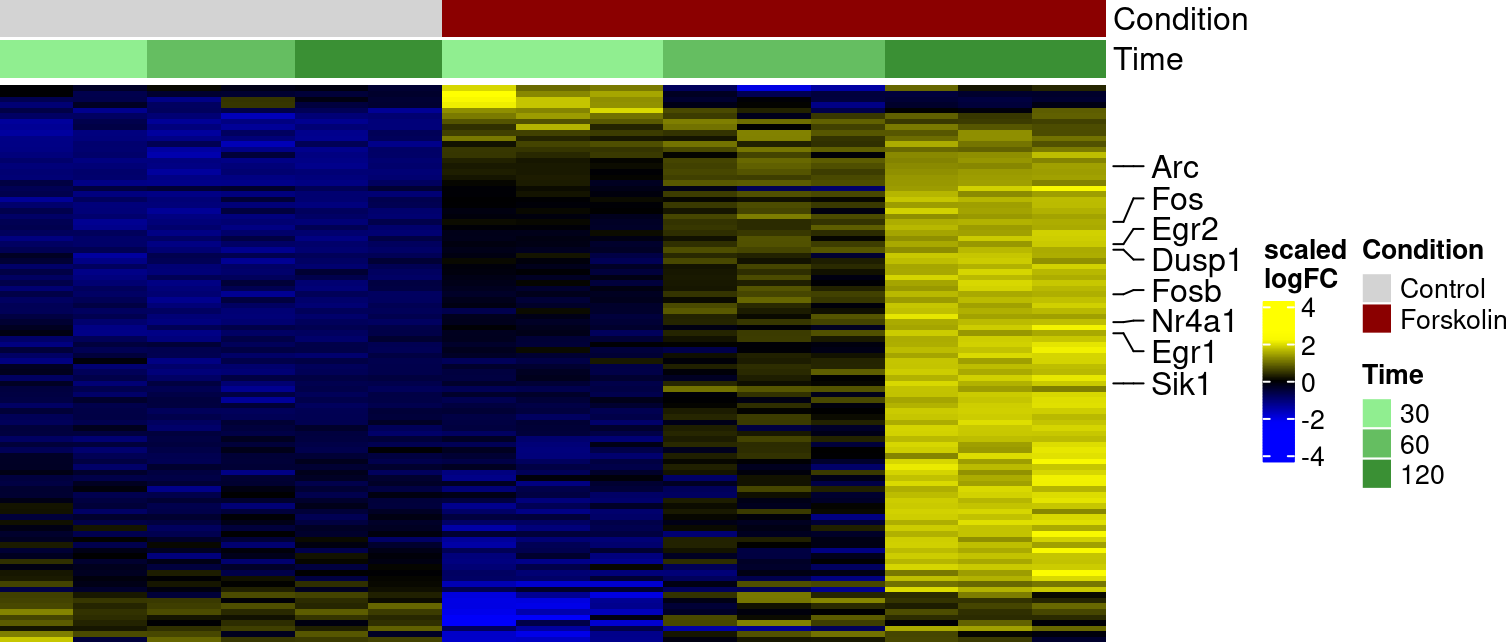We can also add gaps using the same columns:

``sechm(SE, features=g, do.scale=TRUE, top_annotation="Time", gaps_at="Condition")``
``## Using assay logFC``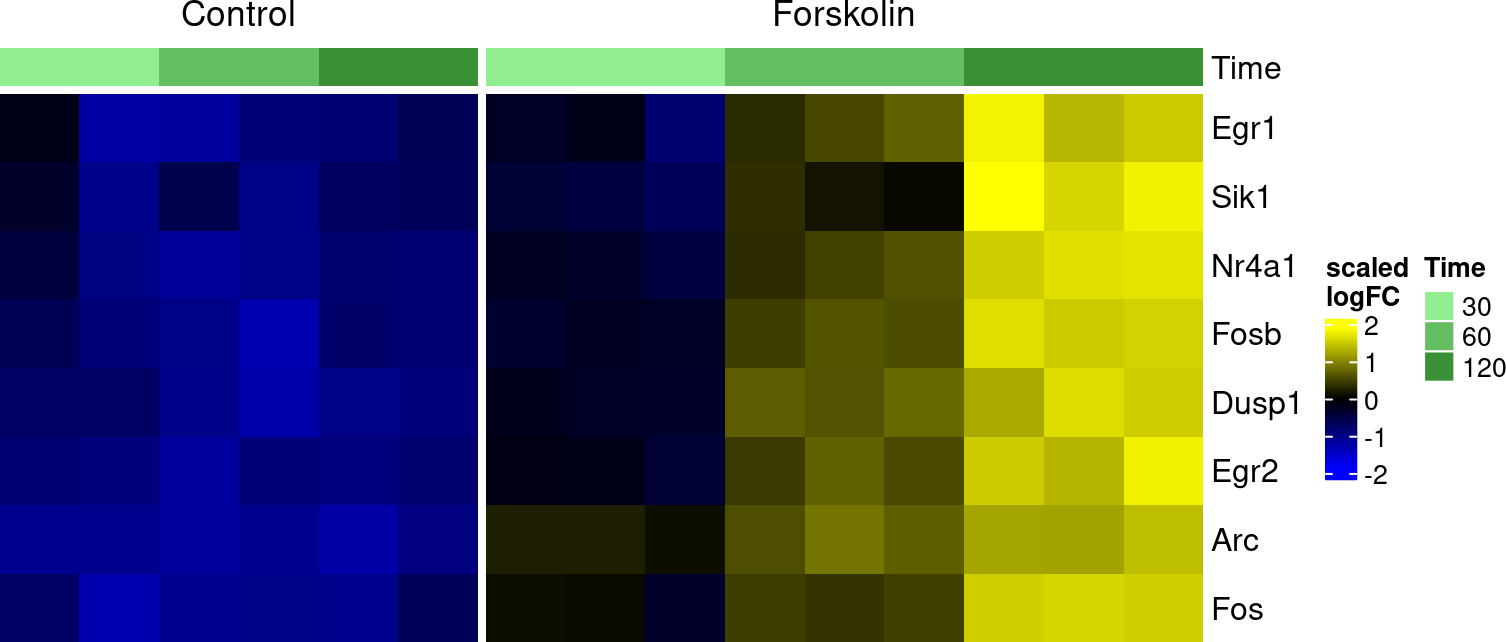## 2.2 Row ordering

By default, rows are sorted using the MDS angle method (can be altered with the `sort.method` argument); this can be disabled with:

``````# reverts to clustering:
sechm(SE, features=row.names(SE), do.scale=TRUE, sortRowsOn=NULL)``````
``## Using assay logFC``